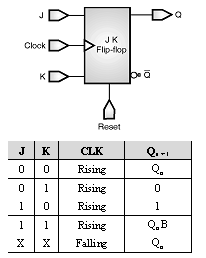Home > VHDL > Flipflops > JKFF

* When you infer a JK flip-flop, make sure you can control the J, K, and clock signals from the top-level design ports to ensure that simulation can initialize the design.

* When you infer a JK flip-flop, make sure you can control the J, K, and clock signals from the top-level design ports to ensure that simulation can initialize the design.

* Example provides the VHDL code that implements the JK flip-flop described in the truth table In the JK flip-flop, the J and K signals act as active high synchronous set and reset. Use
Figure shows the inferred flip-flop.library ieee;

use ieee.std_logic_1164.all;

entity JK_FF is

port (J, K, Clock, Reset : in std_ulogic;

Q, Qbar : out std_ulogic);

end JK_FF;

architecture sig of JK_FF is

signal state : std_ulogic;

begin

process (Clock, Reset) is

begin

if (Reset = '0') then

state <= '0';

elsif rising_edge(Clock) then

case std_ulogic_vector'(J, K) is

when "11" =>

state <= not state;

when "10" =>

state <= '1';

when "01" =>

state <= '0';

when others =>

null;

end case;

end if;

end process;

Q <= state;

Qbar <= not state;

end sig;